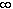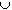#Interactive Real Analysis

Next | Previous | Glossary | Map

## 5.3. Connected and Disconnected Sets

### Proposition 5.3.3: Connected Sets in R are Intervals

If S is any connected subset of R then S must be some interval.

### Proof:

If S is not an interval, then there exists a, bS and a point t between a and b such that t is not in S. Then define the two sets
• U = ( -, t ) and V = ( t,)
Then US # 0 (because it contains { a }) and VS # 0 (because it contains { b }), and clearly (US)(VS) = 0. Finally, because t is not contained in S, we know that (US)(VS) = S. Hence, we have found the required sets U and V to disconnect S. So, we have proved that if a set is not an interval it is disconnected. That is equivalent to saying that if it is connected, it must be an interval.Next | Previous | Glossary | Map